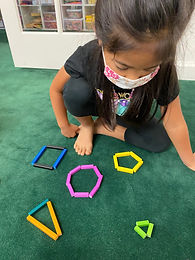## Ms. Brianne

### Target 1​

###### Lesson Type:

New

Number Operation

:

Number Reltionships

Compare equations, recording the results of the comparison with the symbols >, =, and <.

2nd

###### Vocabulary:

Compare, Greater Than, Less Than, Equal to

Activities:

• Students solved and compared addition and subtraction equations using the appropriate inequality symbol.
• Students picked 8 number cards and used them to create their own equations. Then they compared the equations using the symbols >, <, and =.### Home Exploration

###### Guiding Questions:## Absent Students:

### Target 2

:

###### 1:

Classify shapes based on side measurements (2D figures).

###### 2:

Classify shapes as regular (all sides of equal measure).

###### 3:

Classify shapes as irregular (all sides are not of equal measure).

4th

###### Vocabulary:

Side, Angle, Scalene Triangle, Isosceles Triangle, Equilateral Triangle, Right Triangle,

Activities:

• Students created different types of 2D shapes using cuisinaire rods. They identified the type of shape they created based on the number of sides and angles, and if it was regular or irregular.
• Students created the different types of triangles (scalene, isosceles, equilateral, right) and practiced identifying them by name. Then they identified if the triangle was regular or irregular.### Home Exploration

###### Guiding Questions:### Target 3

:

###### Vocabulary:

Activities:### Home Exploration